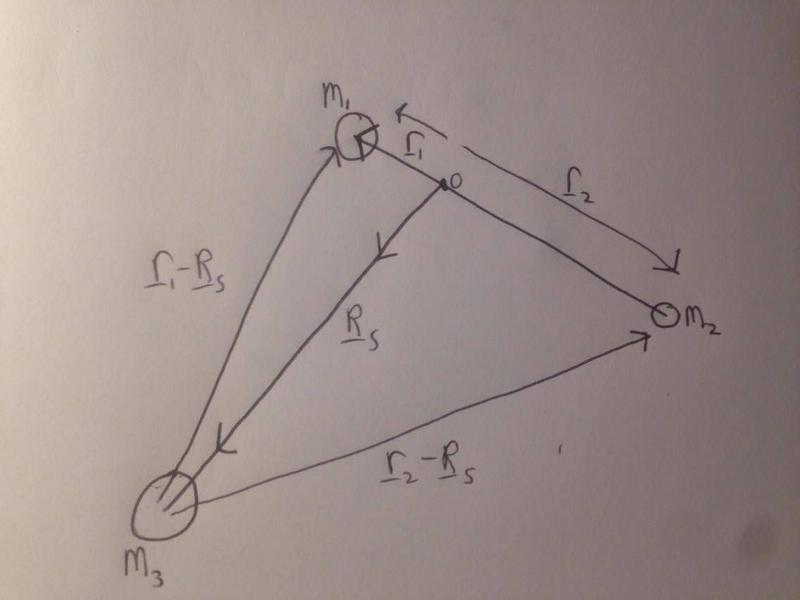# Expressing one Vector in terms of others

## Homework StatementI am very rusty on my mathematics and I am wondering if there is a way to express Rs in terms of r1 and r2. The positions of the bodies are all relative to the origin 0 (C.o.M between m1 and m2). Basically I'm trying to express the two vectors coming from m3 in terms of the masses and position vectors for m1 and m2.

R[/B]3-1 = r1-Rs
R3-2 = r2-Rs

## The Attempt at a Solution

I think r1 and r2 are related through the expression: r2= -(m1/m2)*r1 but this was very much 'fudged' so I think I'm approaching the problem wrong.

Any help much appreciated.
Cheers.